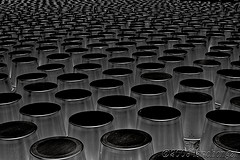## How to get jQuery elements from an array of selectorsTo use an array of strings as jQuery selectors I have found the need to append an empty string.

My bad example works because it coerces the ‘this’ object to a string.

```array = ["h1","p","div"];
\$K(array).each(function() {
console.warn( \$K(this+"").children() );
});
```

Much Better Example:

```array = ["h1","p","div"];
\$K(array).each(function(index, value) {
console.warn( \$K(value).children() );
});
```

I had previously tried the following with out success

```array = ["h1","p","div"];
\$K(array).each(function() {
console.warn( \$K(this).children() );
});
```

The problem of what I was originally trying was that each item in the array wasn’t being treated as a string, I think.The problem of what I was originally trying was that ‘this’ is an object and not a string like I need for the jQuery selector.

A big thanks goes out to my friends,Alex Olson & Sam Curren, for helping me understand my mistake while being tired.

If I didn’t need to do something different for each time through the loop I could do something like this stack overflow answer:

```array = ["h1","p","div"];
console.warn( \$K( array.join(", ") ).children() );
```